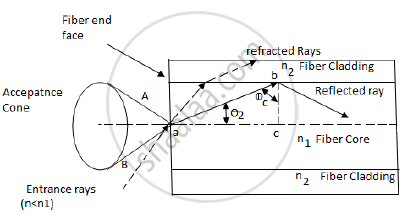# Derive the formula for numerical aperture of step index fibre and give it’s physical significance.The N.A of of an optical fibre is 0.5 and the core refractive index is 1.54. - Applied Physics 2

Derive the formula for numerical aperture of step index fibre and give it’s physical significance.The N.A of of an optical fibre is 0.5 and the core refractive index is 1.54.Find the refractive index of cladding.

#### Solution 1

Numerical Aperture is the ability of fiber to collect the light from the source and save the light inside it by maintaining the condition of total internal reflection.Consider a light ray entering from a medium air of refractive index n0 into the fiber with a core of refractive index n1 which is slightly greater than that of the cladding n2.

Applying Snell’s law of reflection at point A,

(sinӨ_1)/ (sinӨ_2)  = (n_1) / (n_o) = n_1  as  n_0 = 1

In right angled  Δ abc

θ2 = π / 2 − ∅_c

sinθ_1 = n_1sin(π / 2 − ∅_c)

=n_1cos∅_c

cos∅_c = (1− sin∅_c^2)^(1/2)

From the above equation

sinθ1 = n_1(1−sin∅_c^2)^(1/2)

"When the TIR takes place,"  ϕc=θ_c and θ_1=θ_a

sinθ_a = n_1(1−sinθ_c^2)^(1/2)
sinθ_c=n_2/n_1
sinθ_a = n_1[1−((n2)/(n1))^2]^(1/2)
N.A.=sinθ_a
N.A. = sinθ_a = sqrt(n_1^2−n_2^2
Thus,the formula for numerical aperture of step index fibre has been derived.
PHYSICAL SIGNIFICANCE OF NUMERICAL APERTURE :
1.It is the parameter which provides information about the acceptance angle.

2.Smaller N.A is not an advantage because it makes it harder to launch power into fibre.

SOLUTION OF PROBLEM :
N.A. = sqrt(n_1^2−n_2^2)
0.5^2 = 1.54^2 - n_2^2                 n_2 = 1.4566
Refractive index of cladding = 1.4566

#### Solution 2

Numerical Aperture is the ability of fiber to collect the light from the source and save the light inside it by maintaining the condition of total internal reflection.Consider a light ray entering from a medium air of refractive index n0 into the fiber with a core of refractive index n1 which is slightly greater than that of the cladding n2.

Applying Snell’s law of reflection at point A,

(sinӨ_1)/ (sinӨ_2)  = (n_1) / (n_o) = n_1  as  n_0 = 1

In right angled  Δ abc

θ2 = π / 2 − ∅_c

sinθ_1 = n_1sin(π / 2 − ∅_c)

=n_1cos∅_c

cos∅_c = (1− sin∅_c^2)^(1/2)

From the above equation

sinθ1 = n_1(1−sin∅_c^2)^(1/2)

"When the TIR takes place,"  ϕc=θ_c and θ_1=θ_a

sinθ_a = n_1(1−sinθ_c^2)^(1/2)
sinθ_c=n_2/n_1
sinθ_a = n_1[1−((n2)/(n1))^2]^(1/2)
N.A.=sinθ_a
N.A. = sinθ_a = sqrt(n_1^2−n_2^2
Thus,the formula for numerical aperture of step index fibre has been derived.
PHYSICAL SIGNIFICANCE OF NUMERICAL APERTURE :
1.It is the parameter which provides information about the acceptance angle.

2.Smaller N.A is not an advantage because it makes it harder to launch power into fibre.

SOLUTION OF PROBLEM :
N.A. = sqrt(n_1^2−n_2^2)
0.5^2 = 1.54^2 - n_2^2                 n_2 = 1.4566
Refractive index of cladding = 1.4566

Concept: Numerical Aperture
Is there an error in this question or solution?